Learning Library

Probability Toss

5th graders practice probability by using their knowledge of fractions with these probability toss worksheets.

Click on a worksheet in the set below to see more info or download the PDF.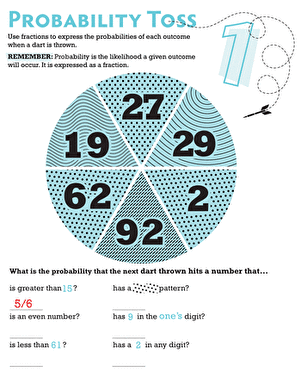Probability Toss 1

What are the chances that a dart will land on an even number on a dart board? Find out in this sixth grade probability worksheet!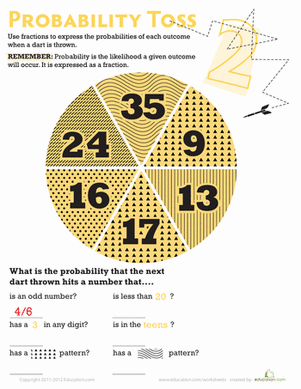Probability Toss 2

Boost your child's math know-how! What are the odds a dart would land on one of the given sections of this circle?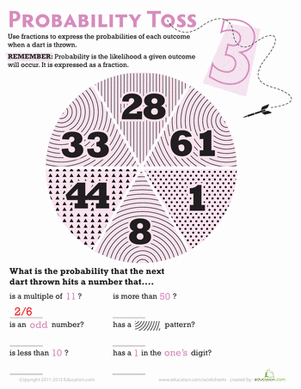Probability Toss 3

If your sixth grader likes darts, then he'll love this probability worksheet! This dart board themed worksheet introduces your child to simple probability.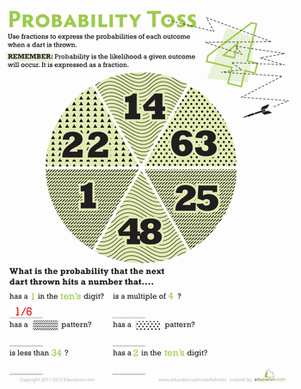Probability Toss 4

Help your sixth grader learn about probability with a dart board math worksheet. Use math skills to calculate the probability of a dart hitting number types.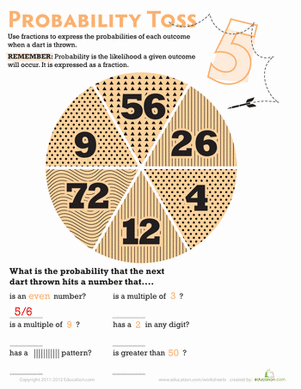Probability Toss 5

Sixth graders will practice probability in this dart board themed worksheet. Your kid will calculate the probability of a dart hitting a certain kind of number.

Want to download this whole set as a single PDF?

Create new collection

0

New Collection>

0 items

What could we do to improve Education.com?

Please note: Use the Contact Us link at the bottom of our website for account-specific questions or issues.

What would make you love Education.com?